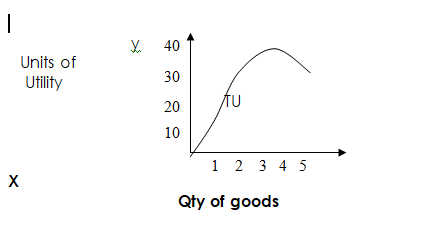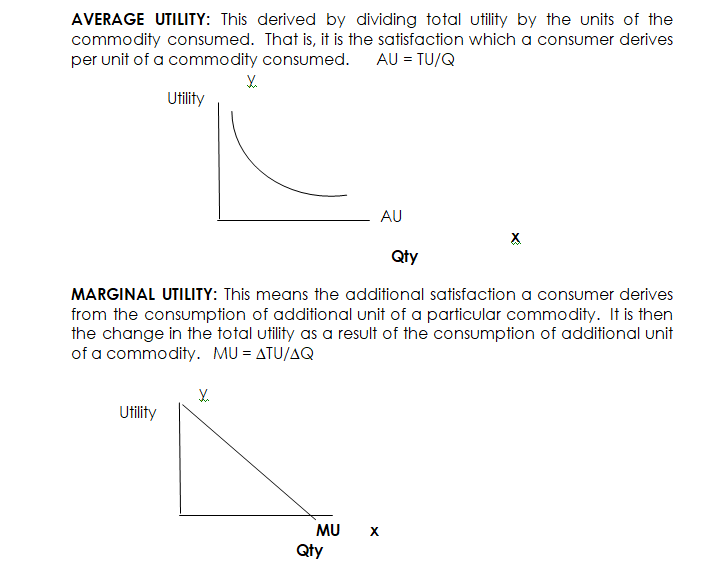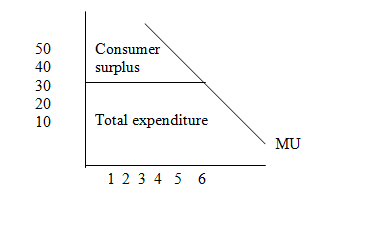# Theory of Consumer Behaviour

Welcome to class!

In today’s class, we will be talking about the theory of consumer behaviour. Enjoy the class!

### THEORY OF CONSUMER BEHAVIOURCONTENT

• Definition of Utility
• Cardinal school of thought/Assumption
• Concept of Total, Average and Marginal Utility
• The law of Diminishing Marginal Utility.

#### THEORY OF CONSUMER BEHAVIOUR

The theory of consumer behaviour is also known as the theory of household behaviour.  It is primarily concerned with how the consumer or household tries to satisfy his/her wants by dividing his/her limited amount of income between the various commodities that give him an equal amount of satisfaction.

##### WHAT IS UTILITY?

Utility can be defined as the satisfaction derived from the consumption of a given commodity.  Hence, when a consumer derives satisfaction from the consumption of a commodity, it can be said that the commodity or service possesses utility.

Utility, therefore, is relative to a consumer, depending on the time, place, form, etc.

A commodity that can satisfy a consumer’s want at a particular point in time and place may not satisfy another’s want.

Evaluation

1. Define utility.
2. What is consumer behaviour?
##### There are basically two schools of thought in the analysis of utility and they are as follows:
1. Cardinal school of thought
2. Ordinal school of thought.
###### CARDINAL SCHOOL OF THOUGHT:

This approach emphasizes that utility is measurable.  That is, after consuming a given quantity of a commodity the consumer can simply evaluate his satisfaction through the use of figures which range from zero to infinity.

ASSUMPTIONS OF CARDINAL APPROACH

1. Utility is measurable
2. The consumer is rational
3. There is diminishing marginal utility
4. Total utility (TU) depends on the quantity consumed.
5. Money income of the consumer is held constant
##### CONCEPT OF TOTAL, AVERAGE AND MARGINAL UTILITY

TOTAL UTILITY: This is the total amount of satisfaction a consumer derives from the consumption of a particular commodity at a point in time.  Consumers’ utility increases as the quantity consumed increases but not at an equal rate because the consumer has a saturation point in the consumption of a particular commodity at a given time.UTILITY SCHEDULE

 Quantity of Goods consumed Total Utility Average Utility Marginal Utility 1 4 4 – 2 7 3.5 3 3 9 3 2 4 10 2.5 1 5 10 2.0 0
##### RELATIONSHIP BETWEEN TOTAL UTILITY AND MARGINAL UTILITY
1. The MU begins to fall right after the first unit of the commodity has been consumed and continues to diminish until it reaches zero level on the x-axis and below.
2. At the point where the MU reaches zero level on the x-axis, TU reaches its maximum point.
3. When the MU cuts the x-axis, TU begins to fall from its peak.
4. When the MU descends below the x-axis and becomes negative, the TU curve begins to slope downward

UTILITY SCHEDULE

 Quantity of Goods consumed Total Utility Average Utility Marginal Utility 1 4 4 – 2 7 3.5 3 3 9 3 2 4 10 2.5 1 5 10 2.0 0Evaluation

1. Calculate the missing value from the table below.
 Quantity of goods consumed Total utility Marginal utility 1 10 – 2 16 A 3 B 4 4 20 C
##### THE LAW OF DIMINISHING MARGINAL UTILITY

The law of diminishing marginal utility states that other things being equal, the marginal utility of a commodity to an individual decrease with an extra unit of that commodity he consumes.  In other words, the law states that if a consumer goes on consuming successive equal increments in the quantity of a commodity, then the increase in total utility resulting will become smaller and smaller, that is, satisfaction per extra unit will start falling.  For instance, a beer drinker may derive maximum satisfaction in the first three bottles, after which decrease in satisfaction may set in as more and more bottles of beer are consumed until he may be unable to consume anymore.

##### UTILITY MAXIMIZATION

Utility maximization is also known as the equilibrium of the consumer.  A point where a consumer derives maximum satisfaction when his marginal utility equates the price of the commodity consumed.  That is, the additional utility derived from the consumption of additional commodity is equal to the price of the commodity.

In the case of one commodity, a consumer will maximize his satisfaction in the consumption of a particular commodity when the MU of that commodity equals the price of that commodity, e.g.  MUx = Px

In the case of two or more commodities, a consumer is said to be in equilibrium or maximizes his utility when the ratio of MU of the last unit of the commodities consumed should be equal to the ratio of the price.  Alternatively, a consumer’s utility is maximized when the MU per amount spent on a product is equal to the MU per amount spent on any other product, as stated below:

MUx             MUy         MUz

Px          =    Py     =        Pz.

where MUx = MU of commodity X

Px    = Price of commodity X

MUy = MU of commodity Y

Py     = Price of commodity Y

MUz = MU of commodity Z

Pz     = Price of commodity Z

##### CONSUMER SURPLUS

Consumer surplus is defined as the difference between the amount a consumer budgeted to pay for a commodity-based on the anticipated level of satisfaction, and the amount he actually paid to have it.  When he consumed the first unit, he was willing to pay as much as #50, but the commodity’s price was #30.  Thus, he saved #20. Therefore, any amount above the market price of #30 represents the consumer’s surplus.###### General evaluation
1. State the law of diminishing marginal utility.
2. Why is scarcity a fundamental problem?
3. Define labour.
4. Differentiate between implicit cost and explicit cost.
5. What is the mobility of labour?
6. List four advantages of the mean

In our next class, we will be talking about Demand and Supply.  We hope you enjoyed the class.

Should you have any further question, feel free to ask in the comment section below and trust us to respond as soon as possible.

How Can We Make ClassNotesNG Better - CLICK to Tell Us💃